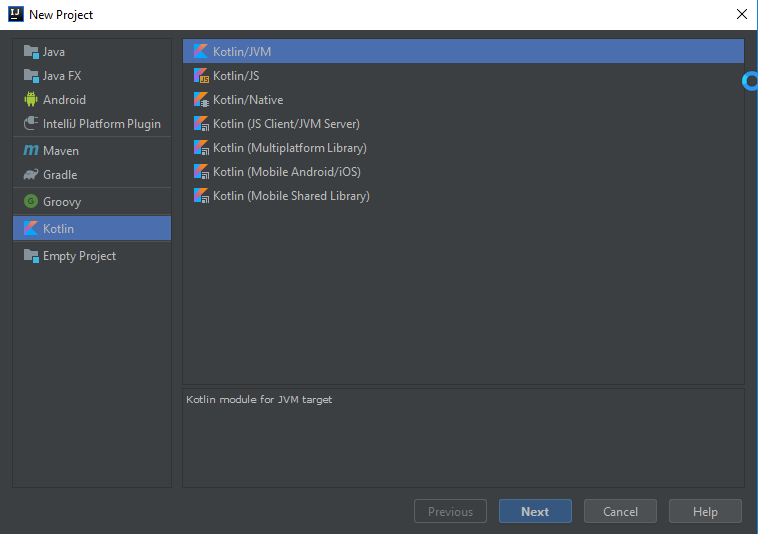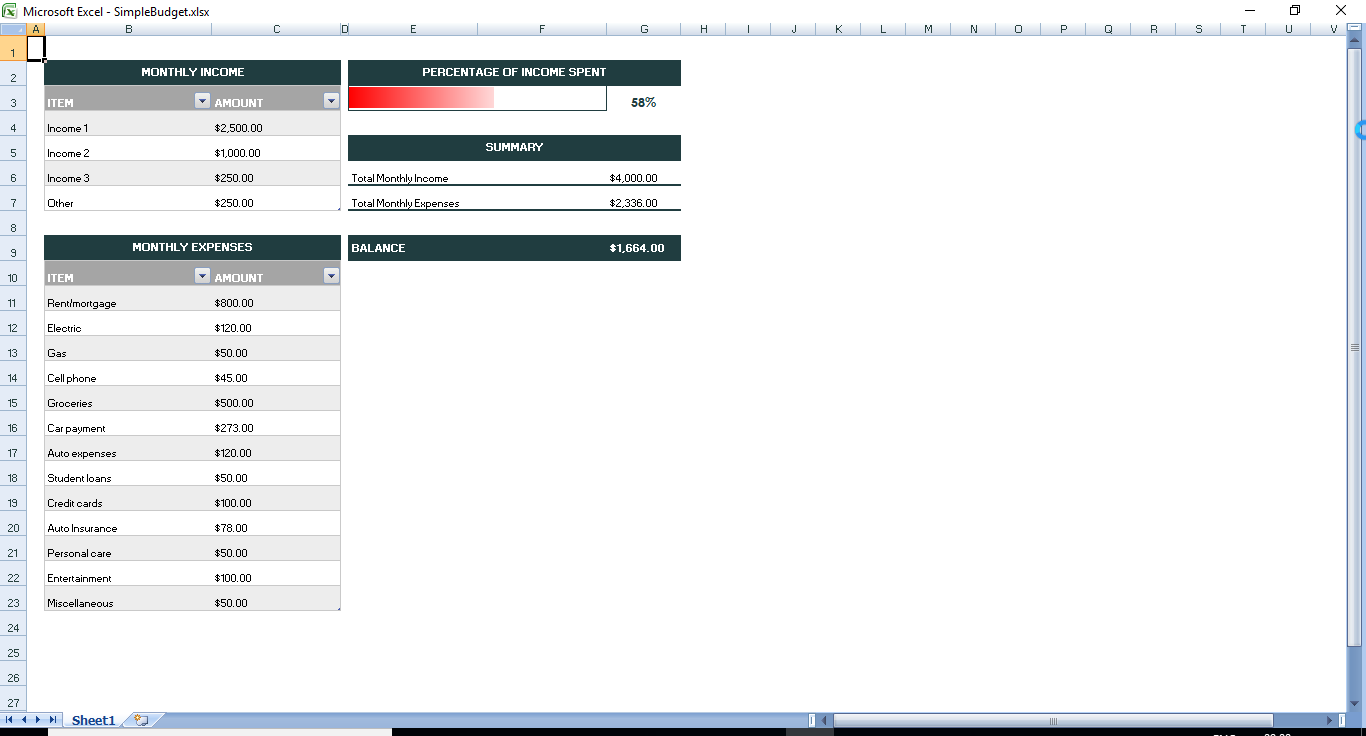﻿ How to Generate Excel Files with Kotlin | GCDocuments# How to Generate Excel Files with Kotlin

Over the past few years, Kotlin is gaining popularity over Java as an alternative language, being as versatile as it's predecessor. A statically-typed programming language running on the Java Virtual Machine (JVM), Kotlin combines both object- oriented and functional programming styles, solving some of the flaws in Java, and is proving to be the most preferred language for Android development.

The main reason to use Kotlin is because it is compatible with Java. All libraries/frameworks from Java can be used in Kotlin, including advanced frameworks. No major steps (like converting projects are required), so one does not have to learn a new language.

Last year, we released GrapeCity Documents for Excel - Java Edition (GcExcel). The library is a high-speed, feature-rich Excel document API based on VSTO that helps to generate, convert, calculate, format, and parse spreadsheets in any application. The feature set helps you work with a variety of features like importing spreadsheets, calculate data, query, generate, and export any spreadsheet, add sorting, filtering, formatting, conditional formatting and validate data, add grouping, sparklines, charts, shapes, pictures, slicers, comments, hyperlinks, and themes.

In addition, you can import existing Excel templates, add data and save the spreadsheets back. You can also use GrapeCity Documents for Excel together with Spread.Sheets, another Spread product that is included in GrapeCity SpreadJS.

Being a Java API, GcExcel is fully compatible with Kotlin. See GcExcel sample browser to view samples running with Kotlin.

### Getting started with GcExcel and Kotlin

#### Step 1:

Open IntelliJ IDE.

#### Step 2:

Create new project.

#### Step 3:

In the left pane, choose 'Kotlin' and in the right pane that opens, choose 'Kotlin/JVM.'

Click Next.#### Step 4:

Give title to the project and click 'Finish.'

#### Step 5:

In the 'src' folder, create 'libs' folder and place gcexcel-2.2.0.jar in it.

#### Step 6:

Right-click 'libs' in the IDE and choose 'Add as library.'

#### Step 7:

In 'src' folder, right-click and add new Kotlin class file, name it 'Main.kt'.

#### Step 8:

Type following code in Main.kt:

a. Import necessary packages:

``````package com.grapecity

import com.grapecity.documents.excel.*
import com.grapecity.documents.excel.drawing.*
import com.grapecity.documents.excel.IWorksheet
import com.grapecity.documents.excel.Workbook
``````

b. Create the main function, add code to generate the workbook and add data to it:

``````fun main(args: Array<String>?) {
val workbook = Workbook()
val worksheet = workbook.worksheets.get(0)

worksheet.getRange("B3:C7").value =
arrayOf(
arrayOf<Any>("ITEM", "AMOUNT"),
arrayOf("Income 1", 2500),
arrayOf("Income 2", 1000),
arrayOf("Income 3", 250),
arrayOf("Other", 250)
)
worksheet.getRange("B10:C23").value =
arrayOf(
arrayOf<Any>("ITEM", "AMOUNT"),
arrayOf("Rent/mortgage", 800),
arrayOf("Electric", 120),
arrayOf("Gas", 50),
arrayOf("Cell phone", 45),
arrayOf("Groceries", 500),
arrayOf("Car payment", 273),
arrayOf("Auto expenses", 120),
arrayOf("Student loans", 50),
arrayOf("Credit cards", 100),
arrayOf("Auto Insurance", 78),
arrayOf("Personal care", 50),
arrayOf("Entertainment", 100),
arrayOf("Miscellaneous", 50)
)

worksheet.getRange("B2:C2").merge()
worksheet.getRange("B2").value = "MONTHLY INCOME"
worksheet.getRange("B9:C9").merge()
worksheet.getRange("B9").value = "MONTHLY EXPENSES"
worksheet.getRange("E2:G2").merge()
worksheet.getRange("E2").value = "PERCENTAGE OF INCOME SPENT"
worksheet.getRange("E5:G5").merge()
worksheet.getRange("E5").value = "SUMMARY"
worksheet.getRange("E3:F3").merge()
worksheet.getRange("E9").value = "BALANCE"
worksheet.getRange("E6").value = "Total Monthly Income"
worksheet.getRange("E7").value = "Total Monthly Expenses"

incomeTable.setName("tblIncome")
incomeTable.setTableStyle(workbook.getTableStyles().get("TableStyleMedium4"))
expensesTable.setName("tblExpenses")
expensesTable.setTableStyle(workbook.getTableStyles().get("TableStyleMedium4"))

worksheet.getRange("E3").setFormula("=TotalMonthlyExpenses")
worksheet.getRange("G3").setFormula("=TotalMonthlyExpenses/TotalMonthlyIncome")
worksheet.getRange("G6").setFormula("=TotalMonthlyIncome")
worksheet.getRange("G7").setFormula("=TotalMonthlyExpenses")
worksheet.getRange("G9").setFormula("=TotalMonthlyIncome-TotalMonthlyExpenses")

worksheet.setStandardHeight(26.25)
worksheet.setStandardWidth(8.43)

worksheet.getRange("2:24").setRowHeight(27.0)
worksheet.getRange("A:A").setColumnWidth(2.855)
worksheet.getRange("B:B").setColumnWidth(33.285)
worksheet.getRange("C:C").setColumnWidth(25.57)
worksheet.getRange("D:D").setColumnWidth(1.0)
worksheet.getRange("E:F").setColumnWidth(25.57)
worksheet.getRange("G:G").setColumnWidth(14.285)

val currencyStyle = workbook.getStyles().get("Currency")
currencyStyle.setIncludeAlignment(true)
currencyStyle.setHorizontalAlignment(HorizontalAlignment.Left)
currencyStyle.setVerticalAlignment(VerticalAlignment.Bottom)
currencyStyle.setNumberFormat("\$#,##0.00")
val percentStyle = workbook.getStyles().get("Percent")
percentStyle.setIncludeAlignment(true)
percentStyle.setHorizontalAlignment(HorizontalAlignment.Center)
percentStyle.setIncludeFont(true)
percentStyle.getFont().setColor(Color.FromArgb(32, 61, 64))
percentStyle.getFont().setName("Century Gothic")
percentStyle.getFont().setBold(true)
percentStyle.getFont().setSize(14.0)
worksheet.getSheetView().setDisplayGridlines(false)
worksheet.getRange("C4:C7, C11:C23, G6:G7, G9").setStyle(currencyStyle)
worksheet.getRange("G3").setStyle(percentStyle)
worksheet.getRange("E6:G6").getBorders().get(BordersIndex.EdgeBottom).setLineStyle(BorderLineStyle.Medium)
worksheet.getRange("E6:G6").getBorders().get(BordersIndex.EdgeBottom).setColor(Color.FromArgb(32, 61, 64))
worksheet.getRange("E7:G7").getBorders().get(BordersIndex.EdgeBottom).setLineStyle(BorderLineStyle.Medium)
worksheet.getRange("E7:G7").getBorders().get(BordersIndex.EdgeBottom).setColor(Color.FromArgb(32, 61, 64))
worksheet.getRange("E9:G9").getInterior().setColor(Color.FromArgb(32, 61, 64))
worksheet.getRange("E9:G9").setHorizontalAlignment(HorizontalAlignment.Left)
worksheet.getRange("E9:G9").setVerticalAlignment(VerticalAlignment.Center)
worksheet.getRange("E9:G9").getFont().setName("Century Gothic")
worksheet.getRange("E9:G9").getFont().setBold(true)
worksheet.getRange("E9:G9").getFont().setSize(11.0)
worksheet.getRange("E9:G9").getFont().setColor(Color.GetWhite())
worksheet.getRange("E3:F3").getBorders().setColor(Color.FromArgb(32, 61, 64))

workbook.save("SimpleBudget.xlsx")
}
``````

#### Step 9:

Run the project.

You have generated an Excel file with Kotlin.### More about GcExcel on Kotlin

To view more demos of GcExcel features with Kotlin, refer to the GcExcel Java sample browser.